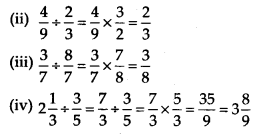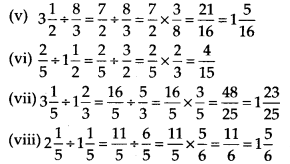# MP Board Class 7th Maths Solutions Chapter 2 Fractions and Decimals Ex 2.4

## MP Board Class 7th Maths Solutions Chapter 2 Fractions and Decimals Ex 2.4

Question 1.
Find: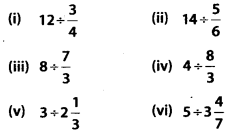Solution: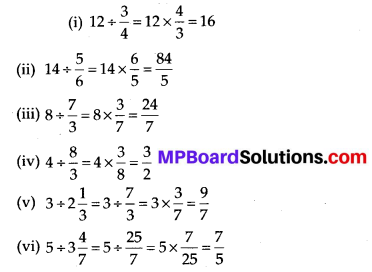Question 2.
Find the reciprocal of each of the following fractions. Classify the reciprocals as proper fractions, improper fractions and whole numbers.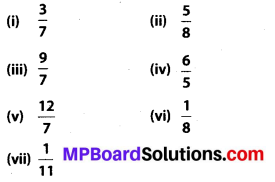Solution:
(i) Reciprocal of $$\frac{3}{7}=\frac{7}{3}$$. It is an improper fraction.
(ii) Reciprocal of $$\frac{5}{8}=\frac{8}{5}$$. It is an improper fraction.
(iii) Reciprocal of $$\frac{9}{7}=\frac{7}{9}$$. It is a proper fraction.
(iv) Reciprocal of $$\frac{6}{5}=\frac{5}{6}$$. It is a proper fraction.
(v) Reciprocal of $$\frac{12}{7}=\frac{7}{12}$$. It is a proper fraction.
(vi) Reciprocal of $$\frac{1}{8}$$ = 8. It is a whole fraction.
(vii) Reciprocal of $$\frac{1}{11}$$ = 11. It is a whole fraction.

Question 3.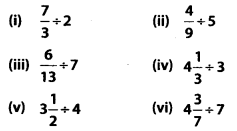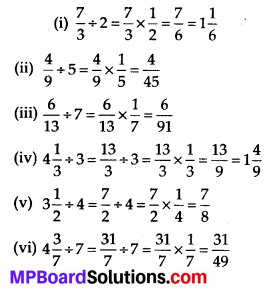Question 4.
Find: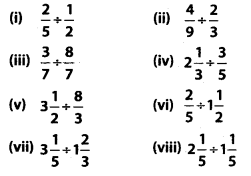Solution:
(i) $$\frac{2}{5}÷\frac{1}{2}=\frac{2}{5} \times 2=\frac{4}{5}$$